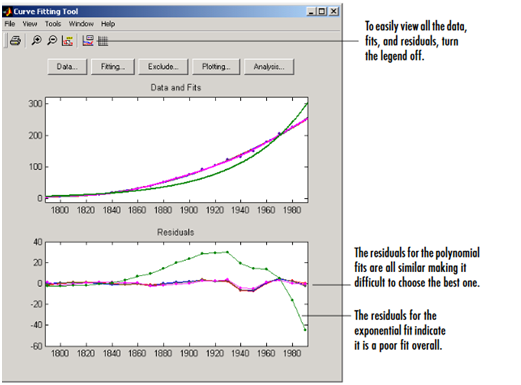# Curve Fitting Homework Help

Searching for curve fitting homework help online? MatlabAssignmentExperts.com provides 100% original curve fitting assignment help by qualified experts.Pocket-friendly price,Flaawless solutions,Excellent Grades,Order Now!

• Curve Fitting Using MATLAB Assignment Help
• What are the differences between the command line and curve-fitting tool environments?
• Avail of our curve fitting using MATLAB homework help if you are not familiar with the procedure of fitting data
• Why should you hire curve fitting in MATLAB tutors at MatlabAssignmentExperts?

## Curve Fitting Using MATLAB Assignment Help

MATLAB boasts of a curve fitting toolbox which consists of graphical user interfaces and M-file functions built on its sophisticated computing environment. This toolbox allows users to do the following:

• Preprocessing of data including smoothing and sectioning
• Data fitting (Parametric fitting is usually done using a toolbox library or custom equation while Non-parametric fitting can be done using various interpolates or a smoothing spline)
• Least squares ( standard linear, non-linear, weighted, constrained) and robust fitting procedures
• Determining the “goodness of fit” using fit statistics

There are two different environments in the curve fitting toolbox in MATLAB:

• The curve fitting tool in the form of a GUI environment
• MATLAB command-line environment

To explore the curve fitting tool, you can simply type cftool. If you are unsure of how to proceed, you can click on the help button on the interface or better still take our curve fitting using MATLAB assignment help. Our eminent Ph.D. qualified experts will work on your project and complete it within the set deadline.

## What are the differences between the command line and curve-fitting tool environments?

These two environments are functionally equivalent. However, it is unacceptable to mix the two when performing a particular curve fitting assignment. For example, you are not allowed to import into the curve fitting tool a fit you have generated at the command line. But a fit can be created in the curve fitting tool and the related M-file generated. After that, the fit can then be recreated from the command line and the M-file modified. It is for this reason that our experts recommend that you use the curve fitting tool for most of your tasks. Also, the curve fitting tool has enhanced data analysis and exploration tools.

## Avail of our curve fitting using MATLAB homework help if you are not familiar with the procedure of fitting data

Data fitting is usually done at the fitting GUI. First, you need to open the fitting graphical user interface by opening the curve fitting tool and clicking on the button “Fitting”. You will notice that the fitting GUI consist of two parts:

1. The Fit Editor

This part allows the user to do the following:

• Specifying the current data set, name of the fit, and the exclusion rule.
• Using a library or custom equation, an interpolant, or a smoothing spline to evaluate whether a variety fits the current data set
• Overriding the default fit options
• Comparing fit results
2. The Table of Fits

This other part of the fitting GUI allows users to do the following:

• Keeping track of the current session’s fits and their data sets
• Producing a detailed fit results summary
• Saving and deleting the results of the fit

The procedure of data fitting can be a hassle. You need an experienced expert in your corner if you are to produce impressive solutions that will convince your professor to award you a straight A. Get our help with curve fitting using MATLAB homework help service and ace your homework. How do you determine the best fit? You should check the numerical and graphical results to determine the best fit. We have explained below how this can be done:

• Examining the graphical fit results

The graphical examination of the fits and residuals should be your initial approach in determining the best fit.For example, in the image above, the graphical fit results indicate that:

• The polynomial equations’ fits and residuals are all similar. This makes it extremely difficult to choose the best one
• An overall poor fit is indicated by the single-term exponential equation’s fit and residuals
1. Examining the numerical fit results

You should examine numerical fit results when you can no longer eliminate fits through graphical examination. The Fitting GUI displays two types of numerical fit results:

• The goodness of fit statistics – It shows if the curve fits the data
• The confidence intervals on the fitted coefficients – It is used to determine the accuracy

For a single fit, you may find some goodness of fit statistics displayed in the Fit Editor’s result area. To enable easy comparison, all goodness of fit statistics are usually displayed in the Table of Fits for all fits.

## Why should you hire curve fitting in MATLAB tutors at MatlabAssignmentExperts?

There are a plethora of reasons why you should hire our curve fitting in MATLAB tutors. Trusting our experts with your project means your task will be handled by professionals who are well-versed in MATLAB. The experts associated with us have prepared several complicated assignments on curve fitting in MATLAB. We guarantee that they are qualified enough to impress you with premium quality solutions. Additionally, our curve fitting in MATLAB experts guarantee:

• Timely delivery of your assignment
• Unlimited free revisions
• 24x7 live support and many more